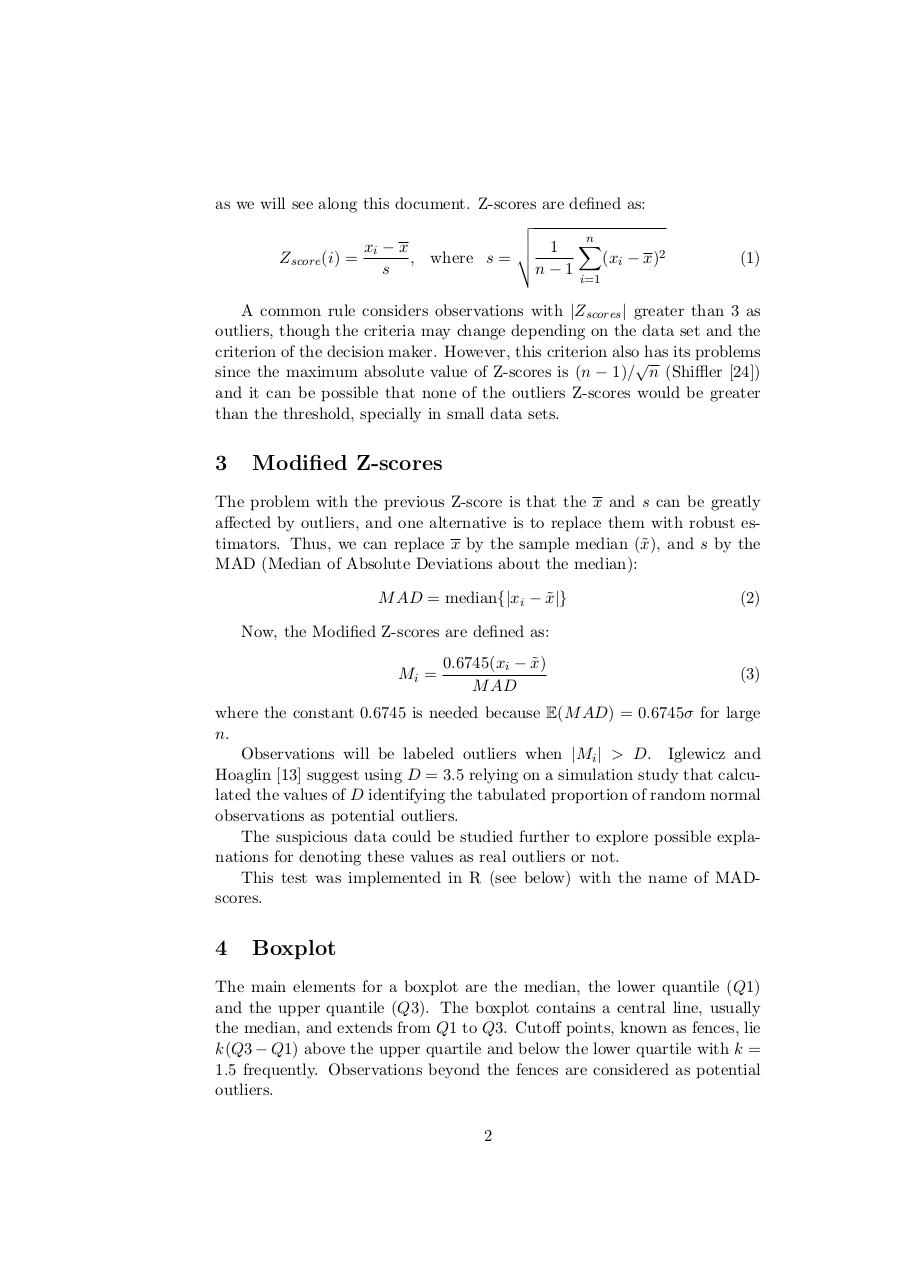# Outlier Methods external.pdfPage 1 23416

#### Text preview

as we will see along this document. Z-scores are defined as:
v
u
n
u 1 X
xi − x
t
Zscore (i) =
, where s =
(xi − x)2
s
n−1

(1)

i=1

A common rule considers observations with |Zscores | greater than 3 as
outliers, though the criteria may change depending on the data set and the
criterion of the decision maker. However, this criterion also has its problems

since the maximum absolute value of Z-scores is (n − 1)/ n (Shiffler )
and it can be possible that none of the outliers Z-scores would be greater
than the threshold, specially in small data sets.

3

Modified Z-scores

The problem with the previous Z-score is that the x and s can be greatly
affected by outliers, and one alternative is to replace them with robust estimators. Thus, we can replace x by the sample median (˜
x), and s by the
M AD = median{|xi − x
˜|}

(2)

Now, the Modified Z-scores are defined as:
Mi =

0.6745(xi − x
˜)

(3)

where the constant 0.6745 is needed because E(M AD) = 0.6745σ for large
n.
Observations will be labeled outliers when |Mi | &gt; D. Iglewicz and
Hoaglin  suggest using D = 3.5 relying on a simulation study that calculated the values of D identifying the tabulated proportion of random normal
observations as potential outliers.
The suspicious data could be studied further to explore possible explanations for denoting these values as real outliers or not.
This test was implemented in R (see below) with the name of MADscores.

4

Boxplot

The main elements for a boxplot are the median, the lower quantile (Q1)
and the upper quantile (Q3). The boxplot contains a central line, usually
the median, and extends from Q1 to Q3. Cutoff points, known as fences, lie
k(Q3 − Q1) above the upper quartile and below the lower quartile with k =
1.5 frequently. Observations beyond the fences are considered as potential
outliers.
2